# How to find the average of an array in JavaScriptself.__wrap_n=self.__wrap_n||(self.CSS&&CSS.supports("text-wrap","balance")?1:2);self.__wrap_b=(e,r,t)=>{t=t||document.querySelector(`[data-br="\${e}"]`);let n=t.parentElement,a=s=>t.style.maxWidth=s+"px";t.style.maxWidth="";let o,i=n.clientWidth,l=n.clientHeight,c=i/2-.25,f=i+.5;if(i){for(a(c),c=Math.max(t.scrollWidth,c);c+1<f;)o=Math.round((c+f)/2),a(o),n.clientHeight===l?f=o:c=o;a(f*r+i*(1-r))}t.__wrap_o||typeof ResizeObserver<"u"&&(t.__wrap_o=new ResizeObserver(()=>{self.__wrap_b(0,+t.dataset.brr,t)})).observe(n)};self.__wrap_n!=1&&self.__wrap_b(":Rj0pm:",1)

November 6, 2023

Doing an average in JavaScript isn’t straightforward. This guide covers how to calculate the average of an array in JavaScript.

## Is there an average method in JavaScript?

No, JavaScript does not have a built-in `average` for arrays. Unlike some programming languages that provide statistical functions as part of their standard libraries, JavaScript's `Array` prototype doesn’t include an `average` function.

To calculate the average of an array in JS, you typically have to manually iterate over the array to sum its elements and then divide the sum by the length of the array. This functionality is often implemented in a custom utility function. Keep reading to learn how to do this.

## Understanding the average

The average, or mean, of an array is a basic statistical measure that represents the central tendency of a set of numbers. It's calculated by summing up all the numbers and then dividing this sum by the total count of numbers.

## Prepare the array

Before you compute the average, ensure that the array contains only numeric values. Non-numeric values can cause errors or incorrect calculations when summing up the elements.

`const numbers = [1, 2, 3, 4, 5]; // An array of numbers`

### Filter non-numeric values

If there's a possibility of non-numeric values in the array, you should filter them out or convert them to a proper numeric form.

```const mixedArray = ['1', null, 2, '3', undefined, 4];
const numericArray = mixedArray.map(Number).filter(n => !isNaN(n));```

## Use a for loop

The most straightforward method to find the average is by using a `for` loop to iterate over the array and sum the elements.

```let sum = 0;
for(let i = 0; i < numbers.length; i++) {
sum += numbers[i];
}
const average = sum / numbers.length;```

## How to find an average using reduce in JavaScript

JavaScript arrays have a `reduce` method which is perfect for accumulating values. This method can simplify the process of summing the array.

```const sum = numbers.reduce((accumulator, currentValue) => accumulator + currentValue, 0);
const average = sum / numbers.length;```

## How to handle empty arrays

When working with arrays, always consider the edge case of an empty array. Attempting to calculate an average from an empty array should be handled gracefully to avoid division by zero.

`const average = numbers.length ? sum / numbers.length : 0;`

## Create a reusable function

For better code reuse and readability, encapsulate the averaging logic within a function.

```function calculateAverage(array) {
const sum = array.reduce((acc, val) => acc + val, 0);
return array.length ? sum / array.length : 0;
}

const average = calculateAverage(numbers);```

## How to deal with arrays of objects

Sometimes the array might consist of objects, and you need to average a specific property.

```const objects = [{ value: 10 }, { value: 20 }, { value: 30 }];

function averageOfProperty(array, key) {
const sum = array.reduce((acc, obj) => acc + (obj[key] || 0), 0);
return array.length ? sum / array.length : 0;
}

const average = averageOfProperty(objects, 'value');```

## Ensure numerical precision

JavaScript's floating-point arithmetic can lead to precision issues. To avoid this, you may need to round the result.

`const preciseAverage = parseFloat(average.toFixed(2));`

### Accurate rounding

A more precise method of rounding is to define a function for it, to handle significant digits correctly.

```function roundPrecisely(number, precision) {
const factor = Math.pow(10, precision);
return Math.round(number * factor) / factor;
}

const roundedAverage = roundPrecisely(average, 2);```

## Performance considerations

For very large arrays, consider performance impacts. Reducing the number of operations, handling computations in chunks, or even utilizing web workers might be necessary.

### How to process large arrays

For arrays with a very large number of elements, use traditional loops instead of methods like `reduce`, which can be less efficient on some JavaScript engines due to function call overhead.

```let sum = 0;
for(let i = 0; i < numbers.length; i++) {
sum += numbers[i];
}
const average = sum / numbers.length;```

### Asynchronous averaging

For non-blocking asynchronous averaging, you can chunk the processing or use Web Workers.

```// This is a conceptual example and not a ready-to-run code
async function calculateAverageAsync(array) {
if (array.length === 0) return 0;

let sum = 0;
let processed = 0;

return new Promise(resolve => {
function processChunk(chunkSize) {
// Process a chunk of the array
for (let i = 0; i < chunkSize && processed < array.length; i++, processed++) {
sum += array[processed];
}
if (processed < array.length) {
// Yield control and schedule the next chunk
setTimeout(() => processChunk(chunkSize), 0);
} else {
// Finish processing
resolve(sum / array.length);
}
}
// Start processing with an initial chunk size
processChunk(1000);
});
}

const averagePromise = calculateAverageAsync(numbers);
averagePromise.then(average => console.log('Average:', average));```

# Ship faster, worry less with Basedash

You're busy enough with product work to be weighed down building, maintaining, scoping and developing internal apps and admin panels. Forget all of that, and give your team the admin panel that you don't have to build. Launch in less time than it takes to run a standup.

You're busy enough with product work to be weighed down building, maintaining, scoping and developing internal apps and admin panels. Forget all of that, and give your team the admin panel that you don't have to build. Launch in less time than it takes to run a standup.

You're busy enough with product work to be weighed down building, maintaining, scoping and developing internal apps and admin panels. Forget all of that, and give your team the admin panel that you don't have to build. Launch in less time than it takes to run a standup.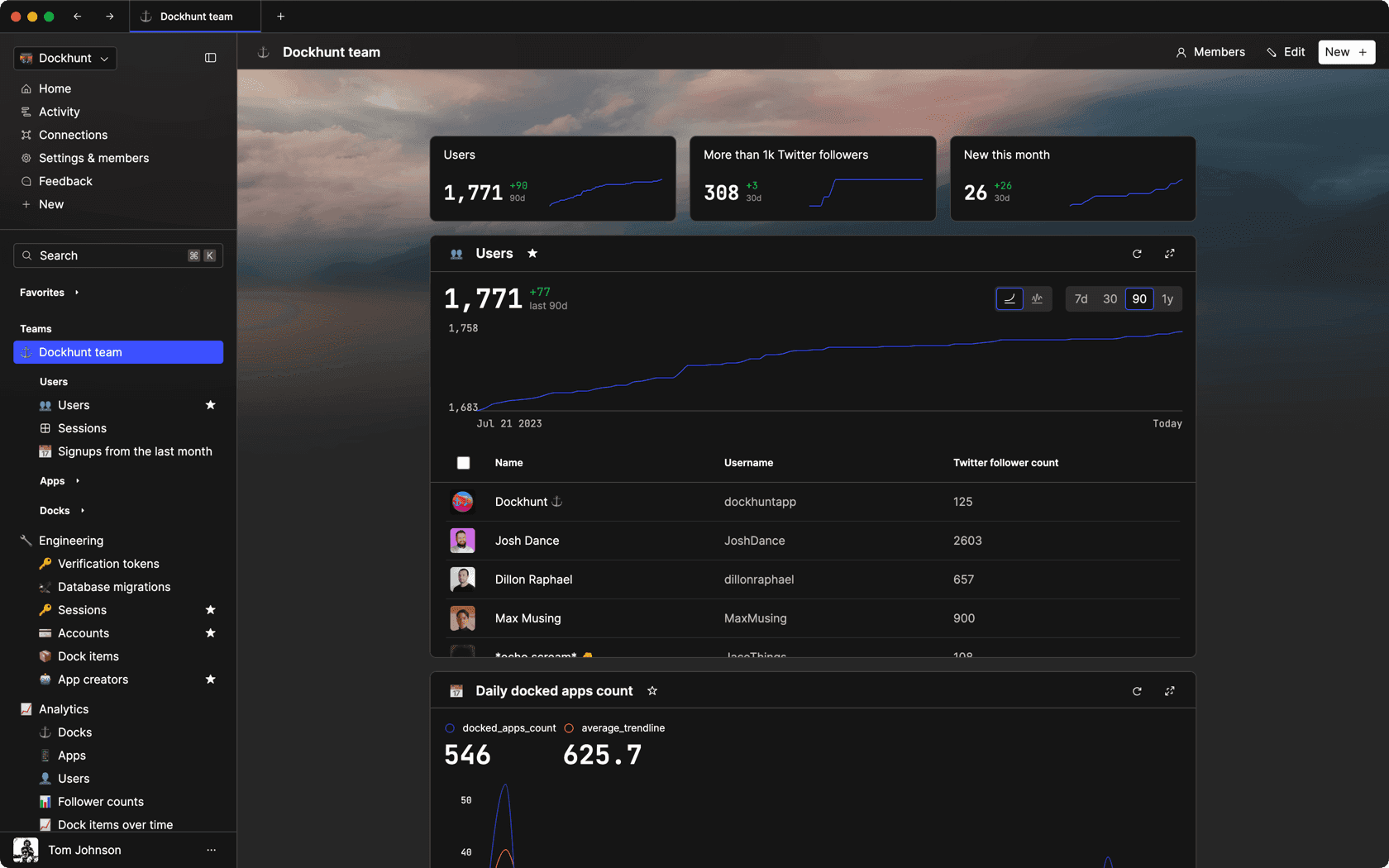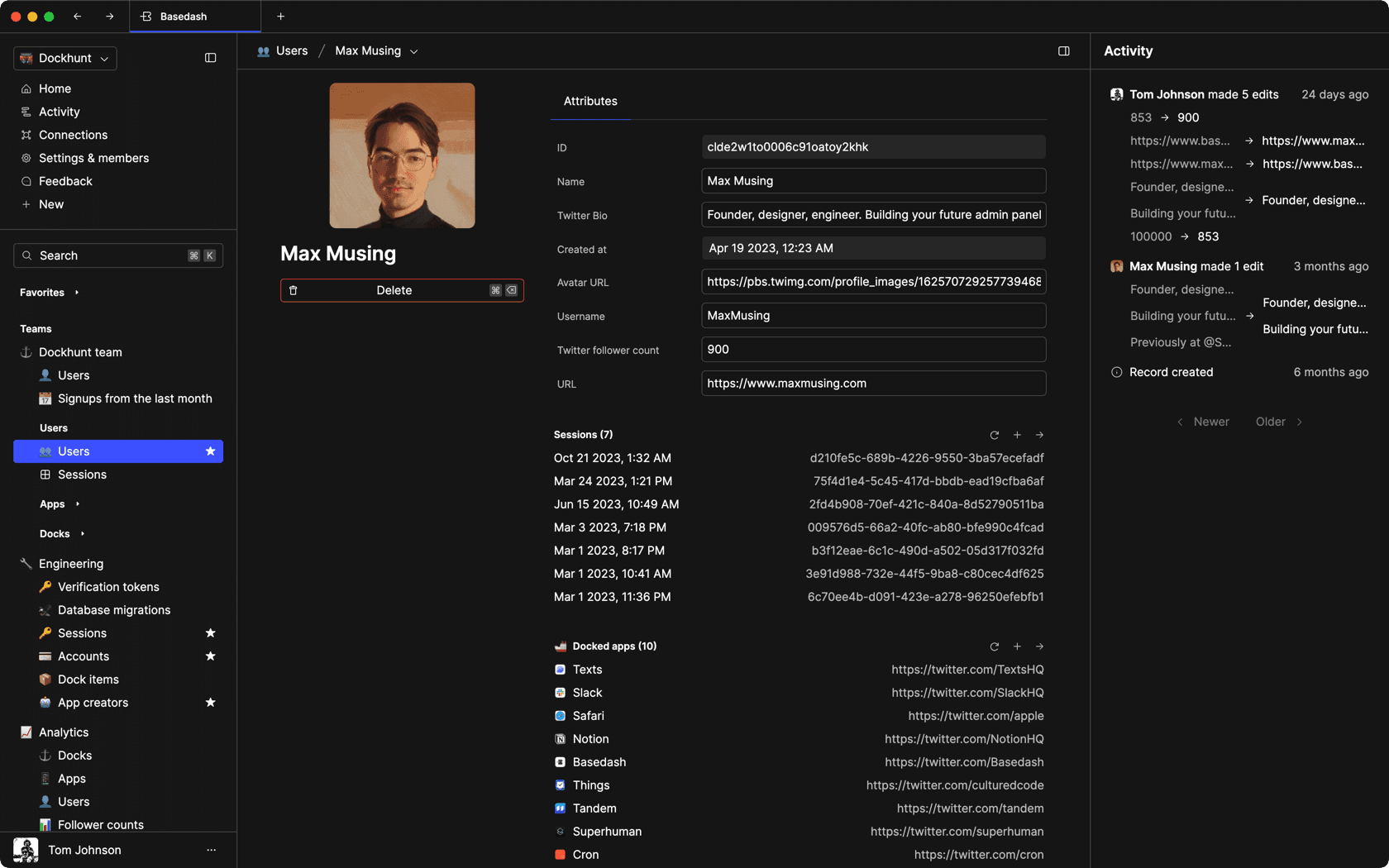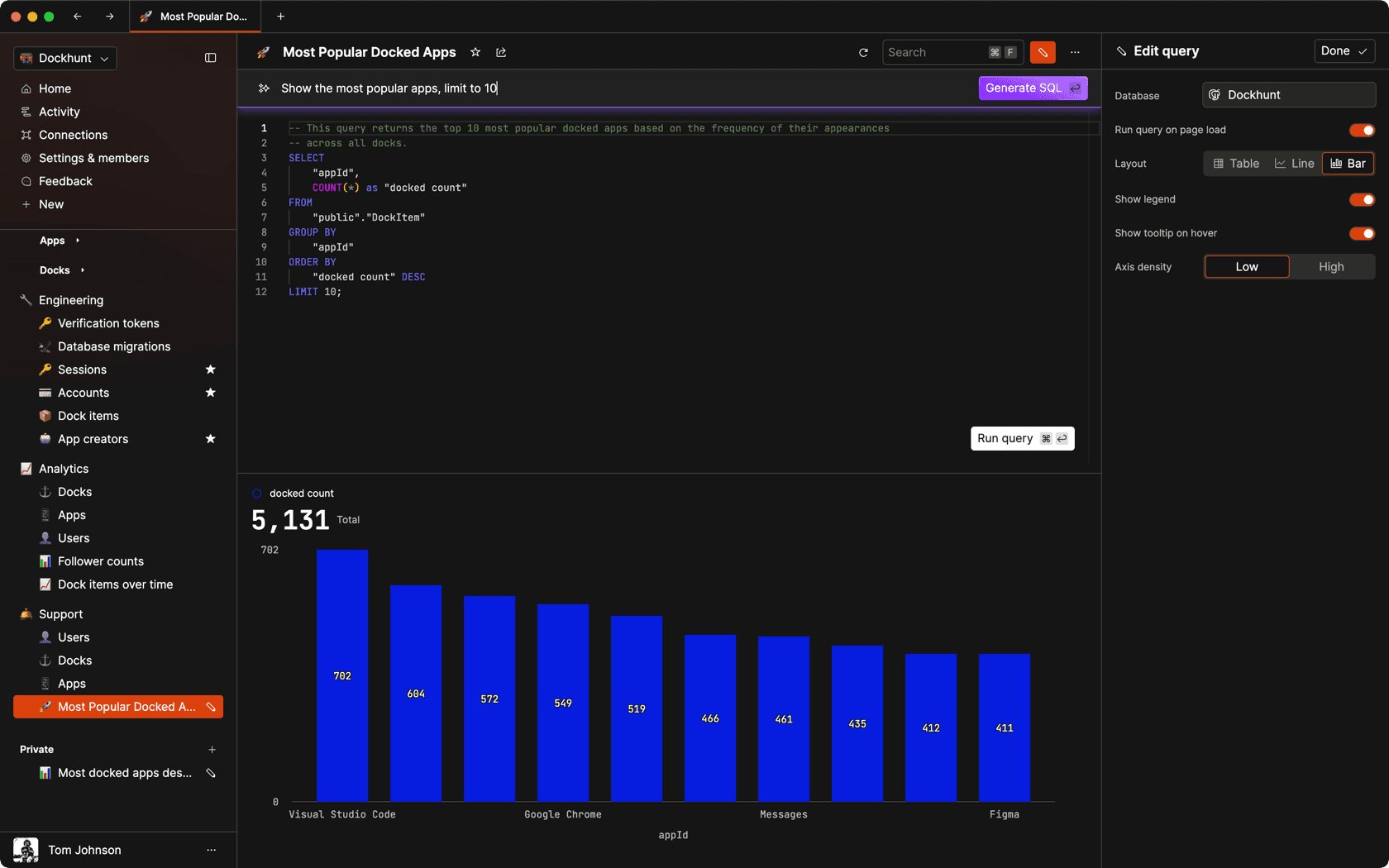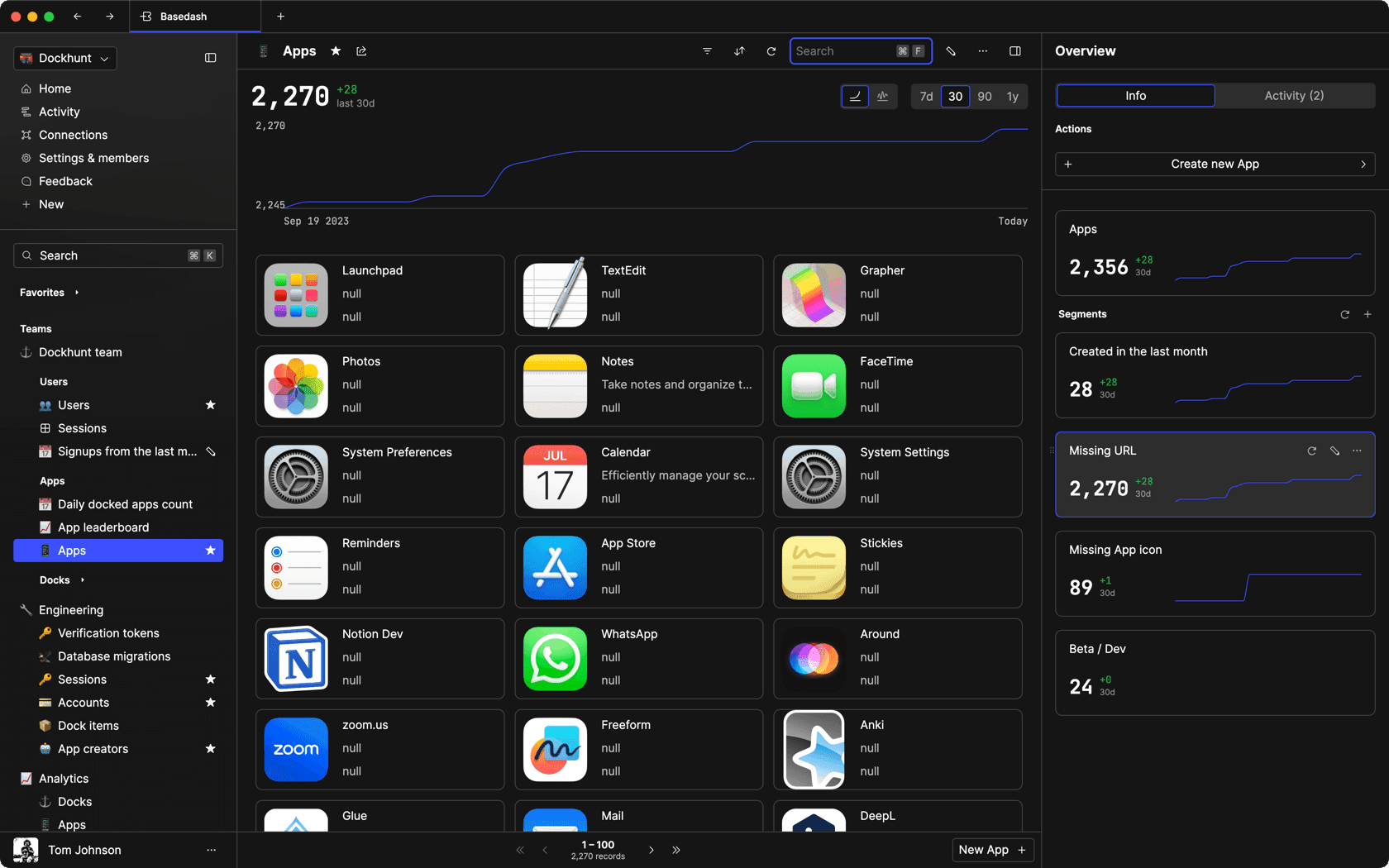### Dashboards and charts

Effortlessly make charts and create a space for your team to work together towards shared goals and metrics.

### SQL composer with AI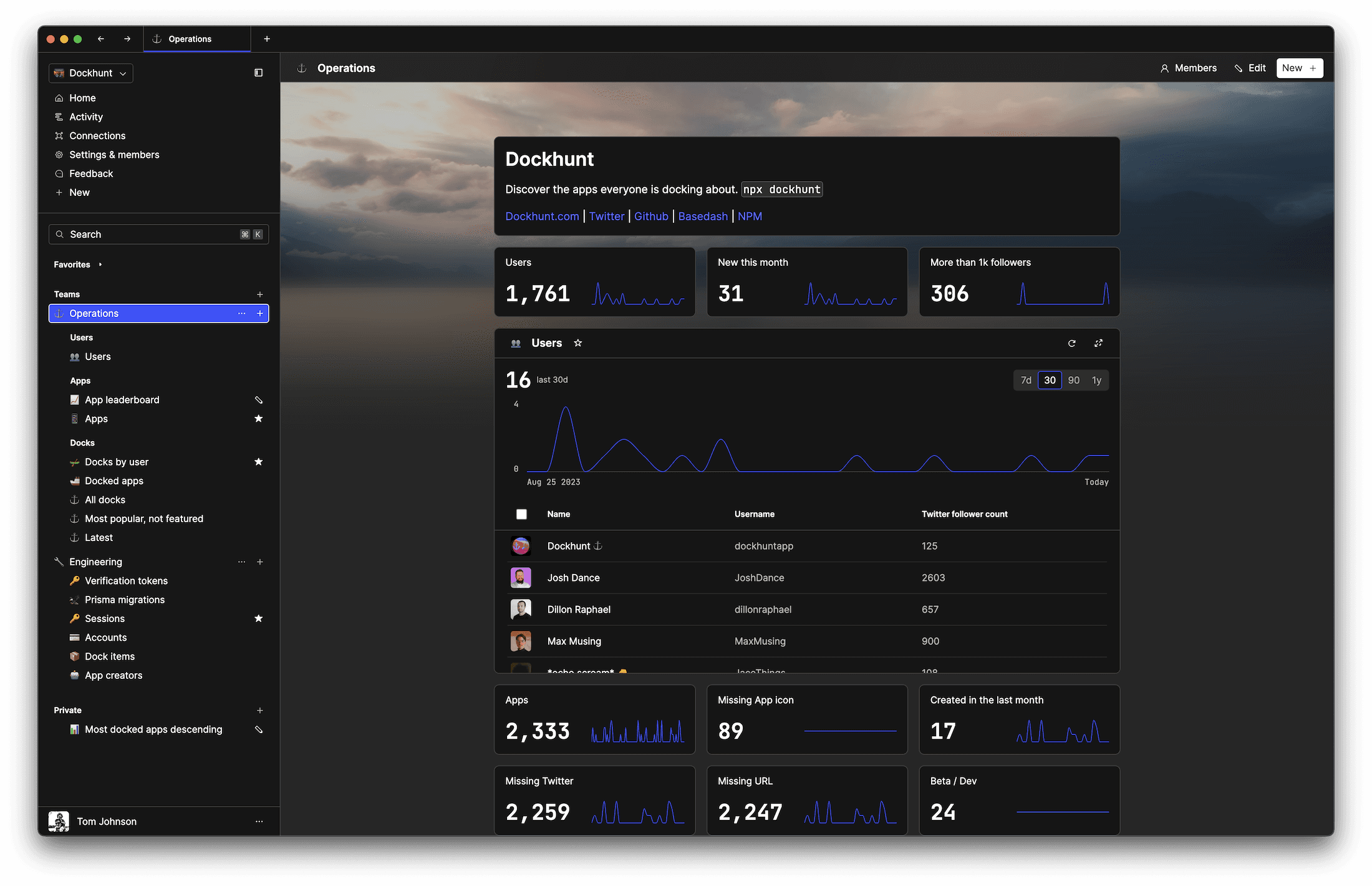SOC II
Type 2 Certified

### Features

Proudly carbon-neutral.SOC II
Type 2 Certified

### Features

Proudly carbon-neutral.SOC II
Type 2 Certified